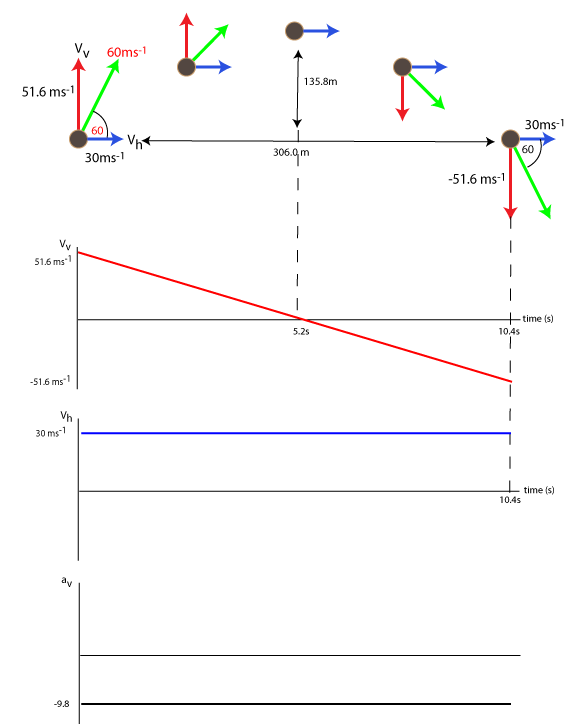Aids to Understanding

Measuring Acceleration

Everyone thinks they can describe how to measure acceleration until they try during an exam. Then they wished the had thought about it more closely. Make sure you don't make that mistake!
Suppose the slope is increased slightly, suggest three different times from the ones in the diagram and calculate a new acceleration that is consistent with a steeper slope.

Graphs of Motion

The pulses of ultrasound are sent out, reflect of car and received. The time between sending and receiving is recorded and the distance from the transmitter/ receiver to car is calculated. If this distances changes then further calculations deduce velocity and acceleration. The software plots these quantities enabling comparison and analysis. In the above example energy losses are ignored.

Graphs of motion 2

Find the velocity and acceleration time graphs for the following motion.

1. A car is travelling at 3.0 ms-1 and accelerates at 2 ms-2 for 3 s.
2. The car travels at a steady speed for 4 s.
3. The car gradually comes to rest with an acceleration of -1.5 ms-2
4. After waiting 9 s, the car accelerates for 2 s at 0.5 ms-2.
5. The car travels at at steady speed for 3 s.
6. The car accelerates at -1 ms-2, until it comes to rest.

Deriving the Equations of Motion

Equation 1 follows from a rearangement of a=(v-u)/t. The authenticity of Equation 4) is self evident ie, the average speed of a body speeding up from u to v with constant acceleration is (v + u)/2, multiplying by t gives us displacement.
To derive Equation 2, we simply draw a velocity time graph starting from u and speeding up to v in time t. The total area under the graph is the displacement of the body during that time.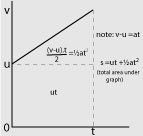To derive equation 2, we start with v = u + at, then square both sides.
We end up with
v2 =u2 + 2uat + a2t2
Grouping terms and taking a common factor of 2a
we end up with
v2 = u2 + 2a(ut + 1/2at2)
v2 = u2 + 2as.

Acceleration is a vector

When treating acceleration as a vector, a negative acceleration does not mean a deceleration and a positive acceleration does not necessarily mean speeding up. The sign indicates direction, ie the negative (left or down) or positive (right or up) direction. For example, the force of gravity always pulls down on a body and given the force and the acceleration it causes must always be in the same direction, the acceleration must also be down, in otherwords, negative. Example 1 a ball is travelling upwards with v = +60.0ms-1, after 3 seconds it is now travelling at = +30.6ms1 Use a = (v-u)/t to calculate the ball's acceleration.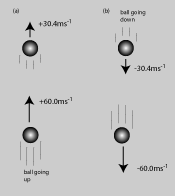Example 2 a ball is travelling downwards with v = -30.6ms-1, after 3 seconds it is now travelling at = -60.0ms1 Use a = (v-u)/t to calculate the ball's acceleration. Compare your answers.

If the sign of a body's velocity and acceleration are the same, the body gets faster. If the sign of a body's velocity and acceleration are not the same, the body slows. The example above verifies this.

Click for homework on Equations of motion

Projectile problems

1) Suppose an astronaut on the moon hits a golf ball at the same angle of elevation and speed as the projectile in the main body text. Complete the exercise and provide a full description of the ball's motion. The Moon's acceleration is -1.6ms-2.
2) A ball is kicked horizontally of a cliff. If the kicker wishes the ball to land avoid 20 m of water below the cliff and the cliff is 30 m high. With what minimum speed will the ball need to leave the kicker's boot?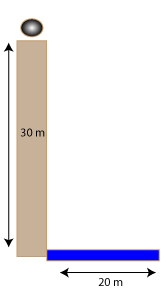## Measuring Acceleration

The Acceleration of a body is defined as a=(v-u)/t.
Suppose a toy car rolls down a slope as shown.
Describe this activity and show that the magnitude of car's acceleration is 0.83 ms-2.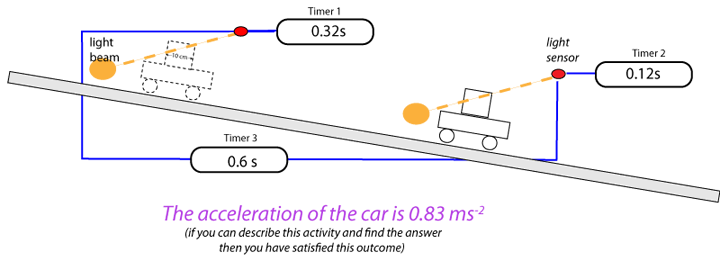## Graphs of Motion

The activity we completed in class tells us all we need to know about drawing velocity-time graphs and
acceleration-time graphs. The positive accelerations of the car indicated on the graph are due to the elastic
band.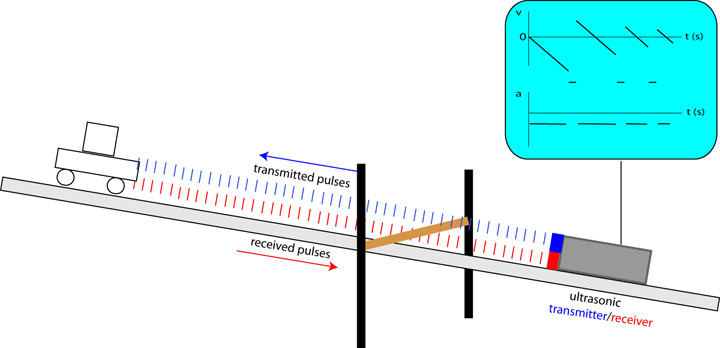## Graphs of Motion 2

This outcome is best illustrated with an example. Consider the six stages of a car's motion.

1. A car is travelling at 5.0 ms-1 and accelerates at 1 ms-2 for 5 s.
2. The car travels at a steady speed for 6 s.
3. The car gradually comes to rest with an acceleration of -1.0 ms-2
4. After waiting 9 s, the car accelerates for 2 sec at -0.5 ms-2.
5. The car travels at at steady speed for 1 second.
6. The car accelerates at 1 ms-2, until it comes to rest.
Draw the a-t and v-t graphs for the motion. The first thing to do is find the total time.

• Stage 1 takes 5 s.
• Stage 2 takes 6s.
• Stage 3 takes 10 s. (Figure out why)
• Stage 4 takes 11s.
• Stage 5 takes 1 s.
• Stage 6 takes 1 s. (Figure out why)
The total time taken is 34 s.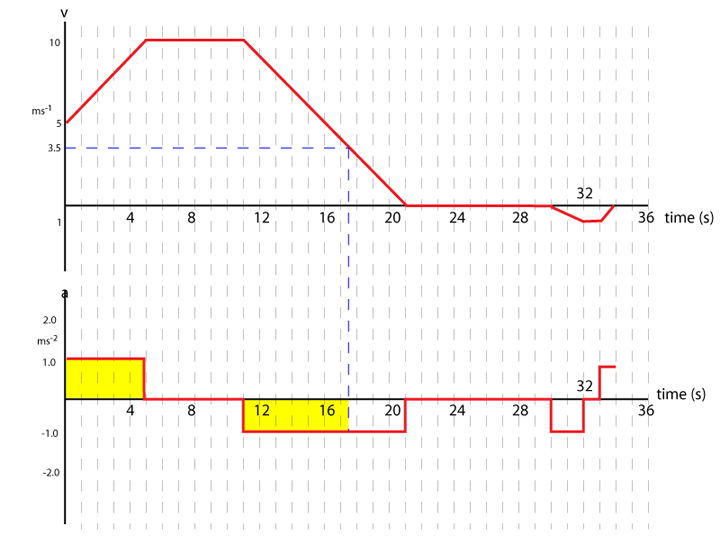Draw this graph yourself, or try to draw the one suggested in the left hand column. Notice that all accelerations are horizontal lines (constant). The area under each graph also has physical significance. The total area under the v-t graph is the displacement of the car (vt =s) and the area under the a-t graph (in yellow) is equal to the change in velocity (at = v - u). Use this information to verify the velocity of the car after 17.5 s is 3.5 ms-1 as indicated by the blue dotted line. Also work out the total area under the a-t graph and relate your value to information on the v-t graph.

## Deriving the Equations of Motion

There are four equations of motion. The equations of motion are normally written for final displacement or final velocity.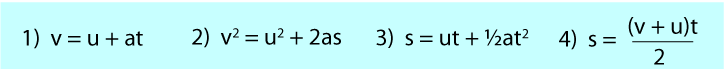1. Equation 1) is for finding the final velocity when we know how long the body has been accelerating for.
2. Equation 2) is for find the final velocity when we know the displacement of the body due to its accelaration.
3. Equation 3) is for finding its final displacement (Note 1) and 3) have identical terms.
4. Equation 4) is simply average velocity x time = displacement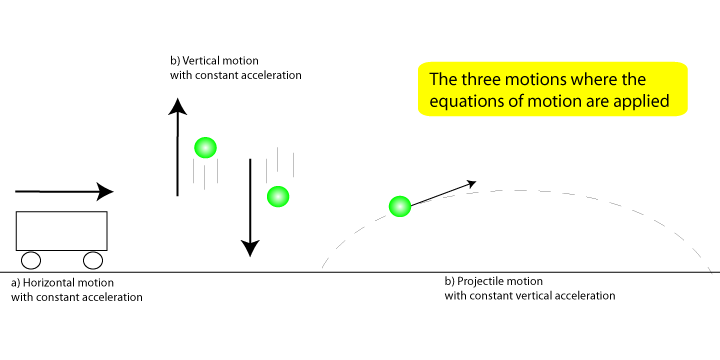## 1) Horizontal motion

Example: A car accelerates at 3.0 ms-2 from rest until it has travelled 10 m.
1. Calculate the car's final velocity. Answer, use equation 2.
2. Calculate the time the car took to travel 10m. Answer use your answer to 1) then use equation 1.
This is the sort of question that could come up. Suppose two runners A and B are running along a track. Runner A has a slower top speed and lesser acceleration than Runner B. However runner A starts a little earlier. The question is normally who wins the race. Example: Two runners are having a race along 150 m track.
• Runner A starts 1 second before runner B.
• Runner A accelerates at 2.0 ms-2 for two seconds, then travels at a steady speed.
• For the final 10 m he decelerates at 0.5 ms-2 until she crosses the line.
• Runner B starts 1 second after runner A.
• Runner B accelerates at 2.2 ms-2 for 2.5 s seconds, then runs at a steady speed.
• For the final 10 m runner B decelerates at 0.4 ms-2 until he crosses the line.
Find the speed of each runner at the crossing line and decide who wins the race. HINT: Draw a velocity-time graph.

An answer to this problem has been provided by Katy an S5 pupil. Katy looked at the graph and quickly concluded B won.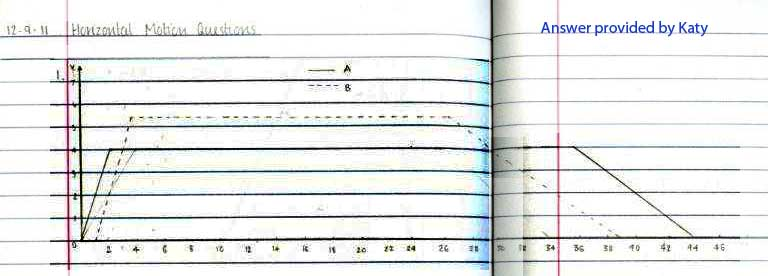A cycle race The green, blue and red teams are in a cycle race. Look very carefully at the diagram and figure out what you could work out here. Your suggestions and solutions will be acknowledged and posted here. Assume the acceleration of the cylcists is constant for the remainder of the race.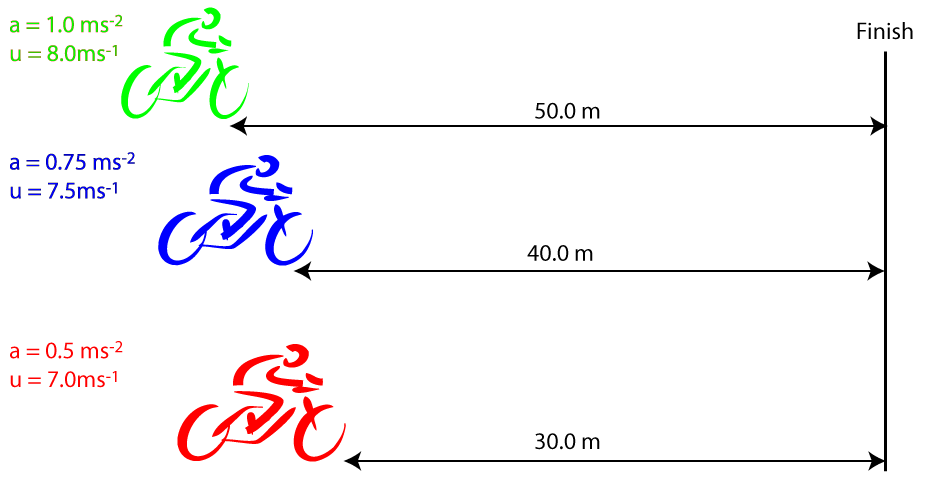## 2) Vertical motion

For bodies going up or coming down their accelerations are always -9.8ms-2. When using -9.8ms-2 you are observing the vectorial nature of acceleration and all velocities and displacements must be given appropriate signs. Up is positive and down is negative.
Example: A hot air ballon is rising above the sea at 5ms-1 and a sandbag is dropped out. If the balloon is 100 m high, how long will it take the sandbag to fall into the sea?. First draw a diagram of the bag's motion.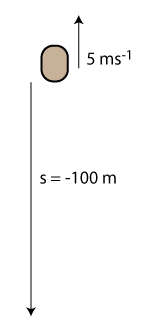Use v2 =u2 + 2as, with a = -9.8ms-2 to find the velocity on impact and then v = u + at to find the time for this change in velocity to take place. Alternatively you can use the quadratic equation s = ut + 1/2at2. However since u is non-zero, you will need to find the roots of a quadratic equation and know how to interpret them.
• v2 =u2 + 2as = v2 = 25 +2(-9.8x-100)
• v = -43.9 ms1
• Now use t = (v-u)/a
• t = (-43.9 - 5)/-9.8
• t = (-48.9)/-9.8 = 4.9s
• The sandbag takes 5s to reach the sea
Note if you choose to ignore the vectorial nature of acceleration and treat a positive acceleration as speeding up and negative acceleration as slowing down then a question like this one has to be treated in two parts and all displacements and velocities are scalars. For this problem, find out how far the bag rises before it stops using a =-9.8ms-2. Add this distance to the 100m and work out how long the bag takes to travel this distance with an acceleration of 9.8ms-2. After this effort you should find treating acceleraton as a vector is simpler and better physics.

## 3) Projectile motion

A projectile can be thought of as a body undergoing vertical and horizontal motion simultaneously. The trajectory below shows the flight of a projectile. The red vectors show the vertical velocity of the projectile at four different times. The blue vectors show the horizontal velocity at four different times. Thus, the vertical velocity continually changes and the horizontal velocity does not change. The green vector indicates the velocity of the projectile.It can be noted that ...
• The vertical velocity vector of a projectile is subject to the force of gravity and equals -9.8ms-2 throughout the flight.
• The horizontal velocity of the projectile remains constant throughout the flight.
• The actual velocity of the projectile is the vector sum of the horizontal and vertical velocities.
• The horizontal and vertical velocities are independent of each other and can be studied separately.
Vertical Information Horizontal Information
sv = uvt + 1/2avt2 sh = uht (note ah = 0)
vv2 = uv2 +2avsv vh2 = uh2 ie vh = uh
vv = uv + avt vh = uh
vv = Vsinq vh = Vcosq
To find the velocity at all points
v2 = vv2 + vh2

## Cumulative question for Equations of Motion

Suppose a projectile is projected at an angle of 60O with a speed of 60ms -1.Since we know the projectile's vertical acceleration is equal to -9.8 ms-2 and its horizontal acceleration is zero, the information provided is sufficient for a complete description of the projectile's path... We can now work out
1. The initial horizontal and vertical components of velocity of the projectile
2. The total time of the flight.
3. The maximum height of the projectile.
4. The maximum range of the projectile.
5. The vertical and horizontal velocities of the projectile at any point during its flight.
6. The vertical and horizontal displacements at any point during the flight.
7. The velocity of the projectile at any point during its flight.
8. The vertical and horizontal velocity-time and acceleration –time graphs for the flight.